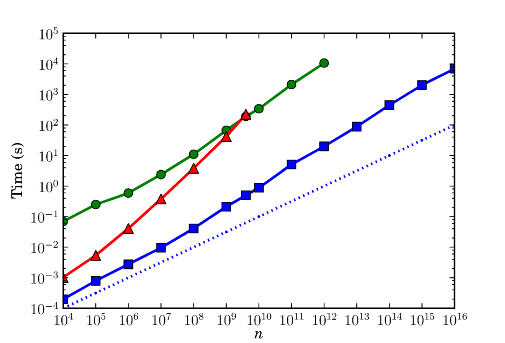# Partitions into the quintillions

March 31, 2012

One of my biggest undertakings last year was to implement the partition function $p(n)$ in FLINT. With this code, I was able to set a record by computing the number of partitions of $10^{19}$, or 10,000,000,000,000,000,000 (ten quintillion).

The number $p(10^{19})$ turns out to have 3,522,804,578 digits, starting and ending with

56469284039962075996762611156427010823552403269435
56912115036797282138529825391237281687215156415242
...
<3522804378 digits omitted>
...
18143637106903146536175827132211646720469971909621
95008234537397439410884324996338596264493674631046


More simply, $p(10^{19}) \approx 5.65 \times 10^{3,522,804,577}$ (this kind of approximation is easy to compute; it is the least significant digits that are challenging — or the more significant digits, for the $p$-adically inclined). This number answers the following practically important combinatorial problem: in how many ways can one choose sticks whose lengths are multiples of one meter, such that they add up to $10^{19}$ meters — approximately the thickness of the Milky Way — when placed end to end?

Okay, computing $p(10^n)$ is just a frivolous benchmark problem. Perhaps more interestingly, at least for number theorists, I generated over 22 billion new Ramanujan-type congruences (identities of the form $p(Ak+B) \equiv 0 \bmod m$ for all $k$) using the algorithm of Rhiannon Weaver. This greatly extends the computation of 76,065 congruences done by Weaver in 2001. An example of a new identity is

$$p(28995244292486005245947069 k + 28995221336976431135321047) \equiv 0 \; \operatorname{mod} \; 29$$

for all values of $k$.

Finding the 22 billion new congruences required evaluating the partition function of approximately 470,000 distinct values up to $n \approx 10^{13}$. This took approximately 150 CPU days distributed over 40-48 cores on a computer at the University of Warwick (access provided courtesy of Bill Hart).

The computation of $p(10^{19})$ took just less than 100 hours of CPU time and roughly 150 GiB of RAM, running on a single core. With 2-3 months of CPU time and 2 TB of swap space, it should be possible to compute the number of partitions of one sextillion ($10^{21}$) with the FLINT code, if anyone is up for the task (unfortunately, parallelization for a single $n$ is not supported, and would be difficult to implement to save more than a factor two).

There are actually three implementations of the partition function in FLINT: one for computing $p(0), p(1), \ldots p(n-1)$, another for computing the same set of values modulo a word-size integer, and finally a function for computing the isolated value $p(n)$. The first two are straightforward applications of FLINT’s fast power series arithmetic, and there is perhaps not that much to say about them (with several gigabytes of RAM, you can comfortably compute perhaps $10^6$ exact values or $10^9$ values modulo a small integer).

The computation of isolated values uses the Hardy-Ramanujan-Rademacher formula

$$p(n)=\frac{1}{\pi \sqrt{2}} \sum_{k=1}^\infty \sqrt{k}\, A_k(n)\, \frac{d}{dn} \left( \frac {1} {\sqrt{n-\frac{1}{24}}} \sinh \left[ \frac{\pi}{k} \sqrt{\frac{2}{3}\left(n-\frac{1}{24}\right)}\right] \right)$$

where

$$A_k(n) = \sum_{0 \le m < k, (m,k) = 1} \,e^{ \pi i \left[ s(m,\, k) \;-\; \frac{1}{k} 2 nm \right]}$$

and $s(m,k)$ denotes a Dedekind sum. This is a numerical infinite series that has to be evaluated approximately. Truncating it appropriately and using a sufficiently high numerical precision gives an approximation that is guaranteed to round to the correct integer.

The FLINT implementation has complexity $O(n^{1/2+\varepsilon})$, which is quasi-optimal since $p(n)$ has about $n^{1/2}$ digits. Implementing the Hardy-Ramanujan-Rademacher formula with this complexity is rather complicated. Ostensibly, there are $n^{3/2}$ terms in the nested sums, and on top of that you need to work with numbers up to $O(n^{1/2})$ digits.

It was only after a lot of digging in the literature that I stumbled across a paper of A. L. Whiteman that gives identities for factoring the exponential sums $A_k(n)$ into short cosine products, reducing the number of terms. This uses quite a bit of modular arithmetic, and implementing it efficiently relies on the fast routines in FLINT for computing gcd, modular square roots, Legendre symbols, factorizations, etc. of word-size integers.

The numerical computations are done using a combination of MPFR arithmetic, some custom routines for high-precision transcendental functions, and machine precision arithmetic for small terms, with carefully managed precision across the whole computation.

Until now, the largest reported computations of $p(n)$ have involved $n$ around $10^9$. Some software certainly allowed going higher than $10^9$, but this was incredibly slow. My code makes it easy to go much higher on commodity hardware, the primary limitation being available memory. For example, computing $p(10^{16})$ with FLINT takes less than two hours on my laptop, using all the available 3 GiB of RAM (plus some swap space).

Compared to the previously best software (Mathematica and Sage), the FLINT code runs around 500 times faster for large $n$. For example $p(2^{32}-1)$ takes half a second in FLINT and around 200 seconds in both Sage and Mathematica.The image is a loglog plot of the time needed to compute $p(n)$ using Mathematica 7 (green circles), Sage 4.7 (red triangles), and FLINT (blue squares). The thin dotted line indicates the slope of the trivial lower complexity bound $\Omega(n^{1/2})$ just for writing out the result. A quick extrapolation suggests that $p(10^{19})$ would take about a decade to compute with Mathematica and a million years with Sage (after patching Sage to allow $n$ larger than 32 bits).

More details about the FLINT partition function implementation (and the computational results) will be given in a forthcoming paper.